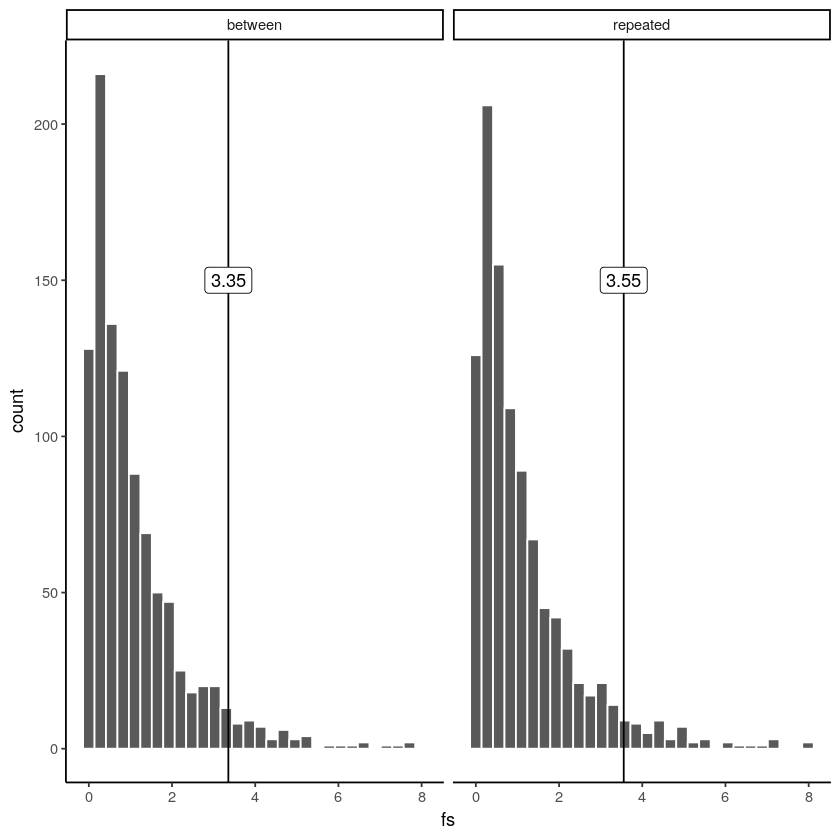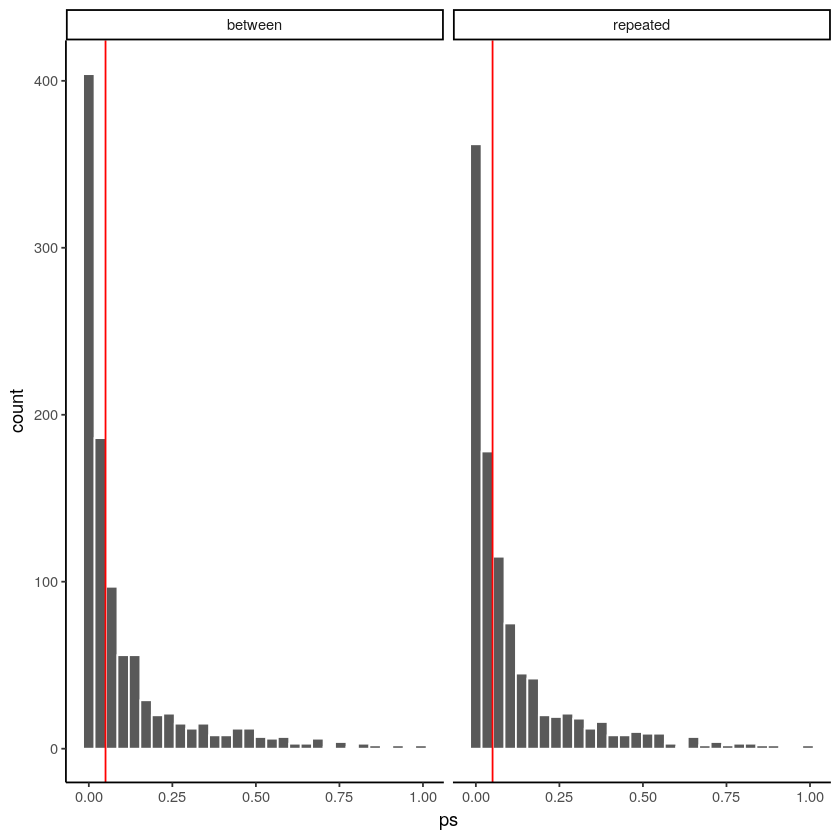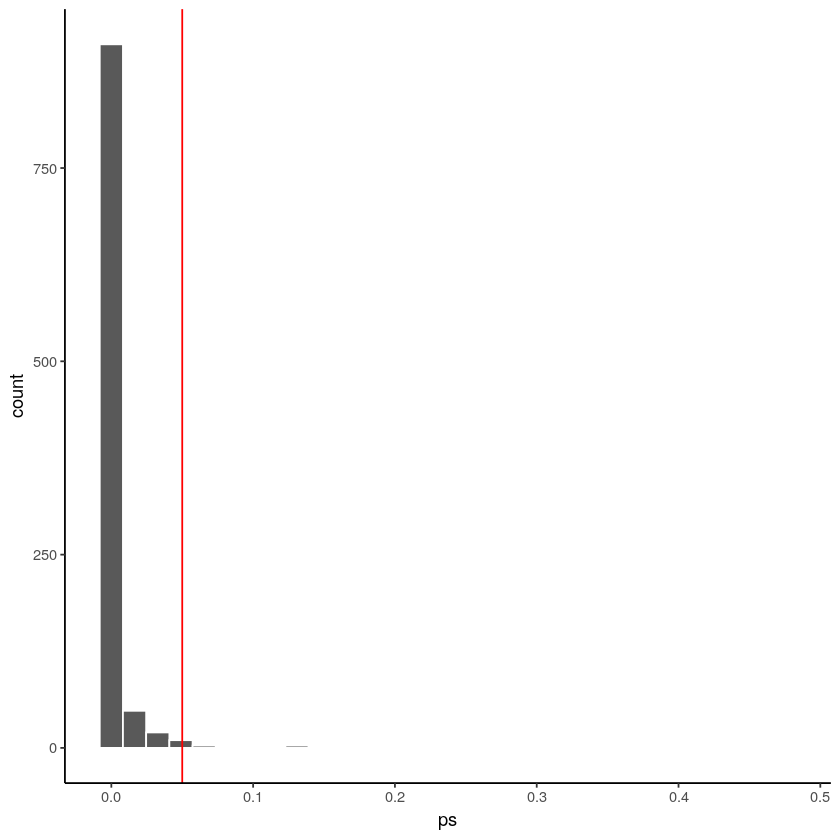# 8.4: Things worth knowing

$$\newcommand{\vecs}{\overset { \rightharpoonup} {\mathbf{#1}} }$$ $$\newcommand{\vecd}{\overset{-\!-\!\rightharpoonup}{\vphantom{a}\smash {#1}}}$$$$\newcommand{\id}{\mathrm{id}}$$ $$\newcommand{\Span}{\mathrm{span}}$$ $$\newcommand{\kernel}{\mathrm{null}\,}$$ $$\newcommand{\range}{\mathrm{range}\,}$$ $$\newcommand{\RealPart}{\mathrm{Re}}$$ $$\newcommand{\ImaginaryPart}{\mathrm{Im}}$$ $$\newcommand{\Argument}{\mathrm{Arg}}$$ $$\newcommand{\norm}{\| #1 \|}$$ $$\newcommand{\inner}{\langle #1, #2 \rangle}$$ $$\newcommand{\Span}{\mathrm{span}}$$ $$\newcommand{\id}{\mathrm{id}}$$ $$\newcommand{\Span}{\mathrm{span}}$$ $$\newcommand{\kernel}{\mathrm{null}\,}$$ $$\newcommand{\range}{\mathrm{range}\,}$$ $$\newcommand{\RealPart}{\mathrm{Re}}$$ $$\newcommand{\ImaginaryPart}{\mathrm{Im}}$$ $$\newcommand{\Argument}{\mathrm{Arg}}$$ $$\newcommand{\norm}{\| #1 \|}$$ $$\newcommand{\inner}{\langle #1, #2 \rangle}$$ $$\newcommand{\Span}{\mathrm{span}}$$

Repeated Measures ANOVAs have some special properties that are worth knowing about. The main special property is that the error term used to for the $$F$$-value (the MSE in the denominator) will always be smaller than the error term used for the $$F$$-value the ANOVA for a between-subjects design. We discussed this earlier. It is smaller, because we subtract out the error associated with the subject means.

This can have the consequence of generally making $$F$$-values in repeated measures designs larger than $$F$$-values in between-subjects designs. When the number in the bottom of the $$F$$ formula is generally smaller, it will generally make the resulting ratio a larger number. That’s what happens when you make the number in the bottom smaller.

Because big $$F$$ values usually let us reject the idea that differences in our means are due to chance, the repeated-measures ANOVA becomes a more sensitive test of the differences (its $$F$$-values are usually larger).

At the same time, there is a trade-off here. The repeated measures ANOVA uses different degrees of freedom for the error term, and these are typically a smaller number of degrees of freedom. So, the $$F$$-distributions for the repeated measures and between-subjects designs are actually different $$F$$-distributions, because they have different degrees of freedom.

## Repeated vs between-subjects ANOVA

Let’s do a couple simulations to see some the differences between the ANOVA for a repeated measures design, and the ANOVA for a between-subjects design.

We will do the following.

1. Simulate a design with three conditions, A, B, and C
2. sample 10 scores into each condition from the same normal distribution (mean = 100, SD = 10)
3. We will include a subject factor for the repeated-measures version. Here there are 10 subjects, each contributing three scores, one each condition
4. For the between-subjects design there are 30 different subjects, each contributing one score in the condition they were assigned to (really the group).

We run 1000 simulated experiments for each design. We calculate the $$F$$ for each experiment, for both the between and repeated measures designs. Here are the two sampling distributions of $$F$$ for both designs.Figure $$\PageIndex{1}$$: Comparing critical F values for a between and repeated measures design.

These two $$F$$ sampling distributions look pretty similar. However, they are subtly different. The between $$F$$ distribution has degrees of freedom 2, and 27, for the numerator and denominator. There are 3 conditions, so $$\textit{df}_{1}$$ = 3-1 = 2. There are 30 subjects, so $$\textit{df}_{2}$$ = 30-3 =27. The critical value, assuming an alpha of 0.05 is 3.35. This means $$F$$ is 3.35 or larger 5% of the time under the null.

The repeated-measures $$F$$ distribution has degrees of freedom 2, and 18, for the numerator and denominator. There are 3 conditions, so $$\textit{df}_{1}$$ = 3-1 = 2. There are 10 subjects, so $$\textit{df}_{2}$$ = (10-1)(3-1) = 92 = 18. The critical value, assuming an alpha of 0.05 is 3.55. This means $$F$$ is 3.55 or larger 5% of the time under the null.

The critical value for the repeated measures version is slightly higher. This is because when $$\textit{df}_{2}$$ (the denominator) is smaller, the $$F$$-distribution spreads out to the right a little bit. When it is skewed like this, we get some bigger $$F$$s a greater proportion of the time.

So, in order to detect a real difference, you need an $$F$$ of 3.35 or greater in a between-subjects design, or an $$F$$ of 3.55 or greater for a repeated-measures design. The catch here is that when there is a real difference between the means, you will detect it more often with the repeated-measures design, even though you need a larger $$F$$ (to pass the higher critical $$F$$-value for the repeated measures design).

## repeated measures designs are more sensitive

To illustrate why repeated-measures designs are more sensitive, we will conduct another set of simulations.

We will do something slightly different this time. We will make sure that the scores for condition A, are always a little bit higher than the other scores. In other words, we will program in a real true difference. Specifically, the scores for condition will be sampled from a normal distribution with mean = 105, and SD = 10. This mean is 5 larger than the means for the other two conditions (still set to 100).

With a real difference in the means, we should now reject the hypothesis of no differences more often. We should find $$F$$ values larger than the critical value more often. And, we should find $$p$$-values for each experiment that are smaller than .05 more often, those should occur more than 5% of the time.

To look at this we conduct 1000 experiments for each design, we conduct the ANOVA, then we save the $$p$$-value we obtained for each experiment. This is like asking how many times will we find a $$p$$-value less than 0.05, when there is a real difference (in this case an average of 5) between some of the means. We will plot histograms of the $$p$$-values:Figure $$\PageIndex{2}$$: p-value distributions for a between and within-subjects ANOVA.

Here we have two distributions of observed p-values for the simulations. The red line shows the location of 0.05. Overall, we can see that for both designs, we got a full range of $$p$$-values from 0 to 1. This means that many times we would not have rejected the hypothesis of no differences (even though we know there is a small difference). We would have rejected the null every time the $$p$$-value was less than 0.05.

For the between subject design, there were 599 experiments with a $$p$$ less than 0.05, or 0.599 of experiments were “significant”, with alpha=.05.

For the within subject design, there were 570 experiments with a $$p$$ less than 0.05, or 0.57 of experiments were “significant”, with alpha=.05.

OK, well, you still might not be impressed. In this case, the between-subjects design detected the true effect slightly more often than the repeated measures design. Both them were right around 55% of the time. Based on this, we could say the two designs are pretty comparable in their sensitivity, or ability to detect a true difference when there is one.

However, remember that the between-subjects design uses 30 subjects, and the repeated measures design only uses 10. We had to make a big investment to get our 30 subjects. And, we’re kind of unfairly comparing the between design (which is more sensitive because it has more subjects) with the repeated measures design that has fewer subjects.

What do you think would happen if we ran 30 subjects in the repeated measures design? Let’s find out. Here we redo the above, but this time only for the repeated measures design. We increase $$N$$ from 10 to 30.Figure $$\PageIndex{3}$$: p-value distribution for within-subjects design with n= 30.

Wowsers! Look at that. When we ran 30 subjects in the repeated measures design almost all of the $$p$$-values were less than .05. There were 982 experiments with a $$p$$ less than 0.05, or 0.982 of experiments were “significant”, with alpha=.05. That’s huge! If we ran the repeated measures design, we would almost always detect the true difference when it is there. This is why the repeated measures design can be more sensitive than the between-subjects design.

8.4: Things worth knowing is shared under a CC BY-SA 4.0 license and was authored, remixed, and/or curated by Matthew J. C. Crump via source content that was edited to conform to the style and standards of the LibreTexts platform; a detailed edit history is available upon request.